# Platonic solid

The Platonic solids (named after the Greek philosopher Plato) are a family of five convex polyhedra which exhibit a particularly high symmetry. They can be characterized by the following two properties: All its sides (faces) are regular polygons of the same shape, and the same number of sides meet in all its corners (vertices). However, they also satisfy the following much stronger symmetry condition: Each flag, i.e. each sequence consisting of a corner of an edge of a face, "looks the same". i.e., cannot be distinguished from the others by its position on the polyhedron. Because of this latter property they are called regular polyhedra.

The Greek names of the Platonic solids are derived from the number of sides:

• Tetrahedron: 4 equilateral triangles, 4 corners in which 3 sides meet
• Hexahedron (or cube): 6 squares, 8 corners in which 3 sides meet
• Octahedron: 8 equilateral triangles, 6 corners in which 4 sides meet
• Dodecahedron: 12 regular pentagons, 20 corners in which 3 sides meet
• Icosahedron: 20 equilateral triangles, 12 corners in which 5 sides meet

Though these names only address the number of sides, they usually are used for the regular (Platonic) solids only.

##  Enumeration

It is easy to see that there are at most five Platonic solids:

The corner of a convex polyhedron is formed by three or more sides, and the sum of the angles cannot exceed 2π.

• Therefore there are only five possibilities: 3, 4, or 5 triangles (angle π/3), 3 squares (angle π/2), and 3 pentagons (angle 3π/5), corresponding to tetrahedron, octahedron, icosahedron, cube, and dodecahedron, respectively.
Of these five possibilities three obviously give polyhedra with the desired properties. For the dodecahedron and the icosahedron, however, additional arguments are needed.
• 6 triangles, 4 squares, and 3 hexagons (angle 2π/3) are special because in these three cases the sum of the angles is 2π, and they give the regular tilings of the plane.
• For n>6, the angle for the regular n-gon is (n-2)π/n, and the sum of three angles is (3-6/n)π and thus is always greater than 2π. Therefore there are no corresponding solids.
• There are two polyhedra with equilateral triangles as sides in addition to the tetrahedron, octahedron and icosahedron. These are a 3-sided and a 5-sided double-pyramid. But they are not Platonic solids since four triangles meet at the base corners, but 3, respectively 5, triangles at the tops of the pyramids.

##  Duality

The central points of the sides of a Platonic solid are also the corners of a Platonic solid (the dual polyhedron):

• The cube and the octahedron are dual to each other.
• The dodecahedron and the icosahedron are dual to each other.
• The tetrahedron is dual to itself.

##  Properties

number
of
faces
name type of face volume surface
area
properties image
4 regular tetrahedron
(or regular triangular pyramid)
equilateral triangle$\tfrac{\sqrt{2}}{12} s^3$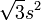$\sqrt{3} s^2$ 4 vertices, 6 edges, self-dual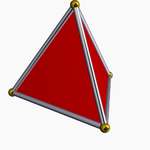6 cube square s3 6s2 8 vertices, 12 edges, dual to octahedron8 regular octahedron equilateral triangle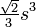$\tfrac{\sqrt{2}}{3} s^3$$2\sqrt{3} s^2$ 6 vertices, 12 edges, dual to cube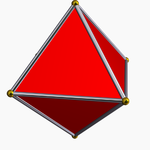12 regular dodecahedron regular pentagon$\tfrac{15+7\sqrt{5}}{4}s^3$$3\sqrt{25+10\sqrt{5}}s^2$ 20 vertices, 30 edges, dual to icosahedron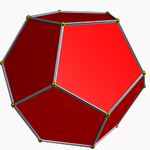20 regular icosahedron equilateral triangle$\tfrac{15+5\sqrt{5}}{12}s^3$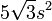$5\sqrt{3}s^2$ 12 vertices, 30 edges, dual to dodecahedronA sphere circumscribed about any of the Platonic solids will touch all the vertices, and a sphere inscribed within any will touch all the faces at the center of the face.Some content on this page may previously have appeared on Citizendium.